# First Order Control System: What is it? (Rise Time, Settling Time & Transfer Function)

Contents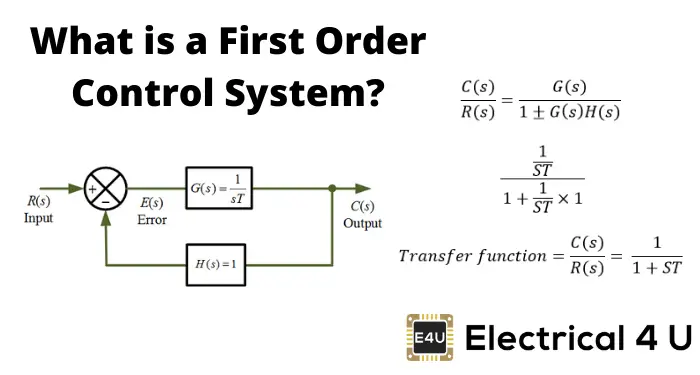## What is a First Order Control System?

A first order control system is defined as a type of control system whose input-output relationship (also known as a transfer function) is a first-order differential equation. A first-order differential equation contains a first-order derivative, but no derivative higher than the first order. The order of a differential equation is the order of the highest order derivative present in the equation.

As an example, let us look at the block diagram of the control system shown below.

The transfer function (input-output relationship) for this control system is defined as: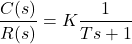Where:

• K is the DC Gain (DC gain of the system ratio between the input signal and the steady-state value of output)
• T is the time constant of the system (the time constant is a measure of how quickly a first-order system responds to a unit step input)

Remember that the order of a differential equation is the order of the highest order derivative present in the equation. We evaluate this with respect to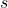.

Since hereis to the first power (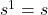), the transfer function above is a first-order differential equation. Hence the block diagram above represents a first-order control system.

In a theoretical alternate example, let us say that the transfer function was equal to: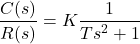In this example sinceis to the second power (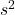), the transfer function is a second-order differential equation. Hence a control system with the above transfer function would be a second-order control system.

Most of the practical models are first-order systems. If a system with higher-order has a dominant first-order mode it can be considered as a first-order system.

Engineers try to find out techniques for systems to become more efficient and reliable. There are two methods of controlling the systems. One is an open-loop control system, and another is a closed-loop feedback control system.

In an open-loop system, the inputs proceed to the given process and produce output. There is no feedback back into the system as for the system to ‘know’ how close the actual output is to the desired output comes.

In a closed-loop control system, the system has the ability to check how far the actual output deviates from the desired output (as the time approaches infinity, this difference is known as the steady state error). It passes this difference as feedback to the controller who controls the system. The controller will adjust its control of the system based on this feedback.

If the input is a unit step, the output is a step response. The step response yields a clear vision of the system’s transient response. We have two types of systems, first-order system, and second-order system, which are representative of many physical systems.

The first order of the system is defined as the first derivative with respect to time and the second-order of the system is the second derivative with respect to time.

A first-order system is a system that has one integrator. As the number of orders increases, the number of integrators in a system also increases. Mathematically, it is the first derivative of a given function with respect to time.

We have different techniques to solve system equations using differential equations or Laplace Transform but engineers have found ways to minimize the technique of solving equations for abrupt output and work efficiency. The total response of the system is the sum of forced response and natural response.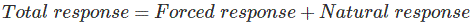The forced response is also called the steady-state response or a particular equation. The natural response is also called the homogeneous equation.

Before proceeding to this topic, you should be aware of the control engineering concepts of poles, zeros, and transfer function and fundamental concepts of the feedback control systems. Here, remind your memory with fundamental concepts of the feedback control system.

## First Order Control System Transfer Function

A transfer function represents the relationship between the output signal of a control system and the input signal, for all possible input values.

### Poles of a Transfer Function

The poles of the transfer function are the value of Laplace Transform variable(s), which cause the transfer function to become infinite.

The denominator of a transfer function is actually the poles of function.

### Zeros of a Transfer Function

The zeros of the transfer function are the values of the Laplace Transform variable(s), that causes the transfer function becomes zero.

The nominator of a transfer function is actually the zeros of the function

## First Order Control System

Here we discuss the first-order control system without zeros. The first-order control system tells us the speed of the response that what duration it reaches the steady-state.

If the input is a unit step, R(s) = 1/s so the output is a step response C(s). The general equation of 1st order control system is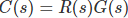, i.e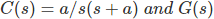is the transfer function.

There are two poles, one is the input pole at the origin s = 0 and the other is the system pole at s = -a, this pole is at the negative axis of the pole plot. We can find the pole and zeros in MATLAB SOFTWARE by using the command pzmap (which stands for pole-zero maps).

We now taking the inverse transform so total response become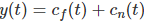which is the sum of forced response and natural response.

Due to the input pole at the origin, produces the forced response as name describe by itself that giving forced to the system so it produces some response which is forced response and the system pole at -a produces a natural response which is due to the transient response of the system.

After some calculation, here General form of the first-order system is C(s) = 1-e-at that is equal to forced response which is “1” and natural response which is equal to “e-at”. The only thing which is needed to find is the parameter “a”.

Many techniques like a differential equation or inverse Laplace Transform, these all solve the total response but these are time-consuming and laborious.

The use of poles, zeros, and there some fundamental concept gives us the qualitative information to solve the problems and due to these concepts, we can easily tell the speed of response and the time of a system to reach the steady-state point.

Let us describe the three transient response performance specifications, the time constant, rise time, and settling time for a first-order control system.

## Time Constant of a First Order Control System

The time constant can be defined as the time it takes for the step response to rise up to 63% or 0.63 of its final value. We refer to this as t = 1/a. If we take reciprocal of time constant, its unit is 1/seconds or frequency.

We call the parameter “a” the exponential frequency. Because the derivative of e-at is -a at t = 0. So the time constant is considered as a transient response specification for a first-order control system.

We can control the speed of response by setting the poles. Because the farther the pole from the imaginary axis, the faster the transient response is. So, we can set poles farther from the imaginary axis to speed up the whole process.

## Rise Time of a First Order Control System

The rise time is defined as the time for the waveform to go from 0.1 to 0.9 or 10% to 90% of its final value. For the equation of rising time, we put 0.1 and 0.9 in the general first-order system equation respectively.

For t = 0.1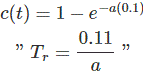For t = 0.9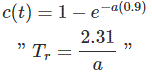Taking the difference between 0.9 and 0.1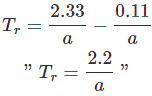Here the equation of rising time. If we know the parameter of a, we can easily find the rise time of any given system by putting “a” in the equation.

## Settling Time of a First Order Control System

The settling time is defined as the time for the response to reach and stay within 2% of its final value. We can limit the percentage up to 5% of its final value. Both percentages are a consideration.

The equation of settling time is given by Ts = 4/a.

By using these three transient response specifications, we can easily compute the step response of a given system that’s why this qualitative technique is useful for order systems equations.

## Conclusion of First Order Control Systems

After learning all things related to 1st order control system, we come to the following conclusions:

• A pole of the input function generates the form of the forced response. It is because of the pole at the origin which generates a step function at the output.
• A pole of the transfer function generates a natural response. It the pole of the system.
• A pole on the real axis generates an exponential frequency of the form e-at. Thus, the farther the pole to the origin, the faster the exponential transient response will decay to zero.
• Using poles and zeros, we can speed up the performance of the system and get the desired output.

Want To Learn Faster? 🎓
Get electrical articles delivered to your inbox every week.
No credit card required—it’s 100% free.